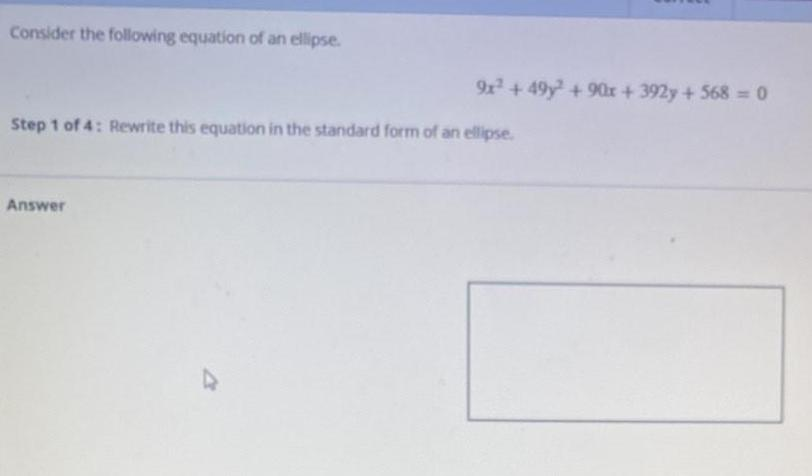Question:

# Consider the following equation of an ellipse. 9x² + 49y²

Last updated: 8/12/2022Consider the following equation of an ellipse. 9x² + 49y² +90x+ 392y + 568 = 0 Step 1 of 4: Rewrite this equation in the standard form of an ellipse. Answer